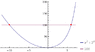# Log problem (or by whatever method)

• tony24810

## Homework Statement

a^2 + 2^a = 100, where a is an integer, find a.

## Homework Equations

all laws of indices and laws of log, I think

## The Attempt at a Solution

By trial and error, answer can be easily determined, which is 6.

However, I am unsure how to approach this problem with algebraic approach. My attempts with log basically fail, because i ended up with log (a+b), which I don't know how to carry on.

I don't think this can be solved algebraically (at least, with "elementary" algebra). I found a second solution graphically (graph y = x2 + 2x and y = 100 on a graphing calculator and find where the two graphs intersect). The second solution is not an integer.

Didn't get a solution too...Tried it on wolframalpha: http://www.wolframalpha.com/input/?t=crmtb01&f=ob&i=a^2+2^a=100
Solutions are: 6 and -9.99995(approx.)
Graph's attached.

#### Attachments

•MSP14211cc39ffdh93116cf00003929503i22018c3g.gif
3.4 KB · Views: 327

## Homework Statement

a^2 + 2^a = 100, where a is an integer, find a.

## Homework Equations

all laws of indices and laws of log, I think

## The Attempt at a Solution

By trial and error, answer can be easily determined, which is 6.

However, I am unsure how to approach this problem with algebraic approach. My attempts with log basically fail, because i ended up with log (a+b), which I don't know how to carry on.

Logs will not help. If 'a' is an integer, it must be a positive integer (can you see why?), so there are only a few possibilities, and you can easily try them out.

For positive integer a, the largest number of the form 2^a that is less than 100 is 64 = 2^6. You can test whether a = 6 solves the problem. If not, try a = 5, then a = 4, etc. Note that this method is not really 'trial and error'; it uses logic to cut the possibilities down to a small number.

Logs will not help. If 'a' is an integer, it must be a positive integer (can you see why?), so there are only a few possibilities, and you can easily try them out.

For positive integer a, the largest number of the form 2^a that is less than 100 is 64 = 2^6. You can test whether a = 6 solves the problem. If not, try a = 5, then a = 4, etc. Note that this method is not really 'trial and error'; it uses logic to cut the possibilities down to a small number.

If 'a' is an integer, it must be a positive integer (can you see why?)

As for your question, I figured that 2^(-ve integer) gives decimals, so no (-ve integer)^2 would always give integer, thus they never add up to 100, is that what you mean?